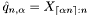#Boost C++ Libraries

...one of the most highly regarded and expertly designed C++ library projects in the world.

This is the documentation for an old version of Boost. Click here to view this page for the latest version.

## Struct template tail_quantile_impl

boost::accumulators::impl::tail_quantile_impl — Tail quantile estimation based on order statistics (for both left and right tails).

## Synopsis

```// In header: <boost/accumulators/statistics/tail_quantile.hpp>

template<typename Sample, typename LeftRight>
struct tail_quantile_impl {
// types
typedef Sample result_type;

// construct/copy/destruct
tail_quantile_impl(dont_care);

// public member functions
template<typename Args> result_type result(Args const &) const;
};```

## Description

The estimation of a tail quantilewith levelbased on order statistics requires the chaching of at least thesmallest or thelargest samples,being the total number of samples. The largest of thesmallest samples or the smallest of thelargest samples provides an estimate for the quantile:

Equation 1.16.### `tail_quantile_impl` public construct/copy/destruct

1. `tail_quantile_impl(dont_care);`

### `tail_quantile_impl` public member functions

1. `template<typename Args> result_type result(Args const & args) const;`